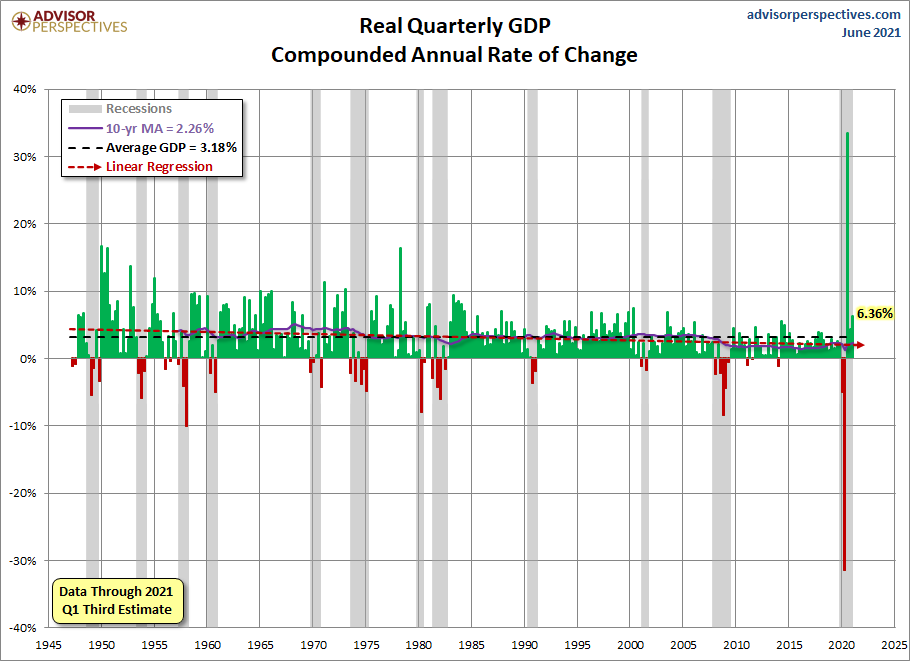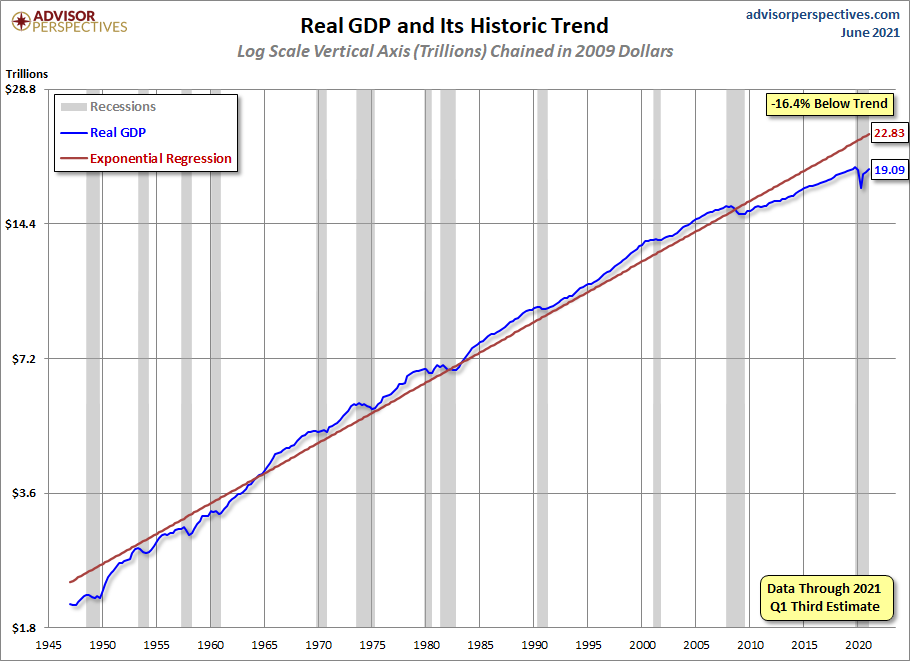## Q1 GDP Third Estimate: Real GDP Remains At 6.4%

The Third Estimate for Q1 GDP, to one decimal, came in at 6.4% (6.36% to two decimal places), an increase from 4.3% (4.33% to two decimal places) for the Q4 Third Estimate. Investing.com had a consensus of 6.4%.

Here is the slightly abbreviated opening text from the Bureau of Economic Analysis news release:

Real gross domestic product (GDP) increased at an annual rate of 6.4 percent in the first quarter of 2021 (table 1), according to the "third" estimate released by the Bureau of Economic Analysis. In the fourth quarter, real GDP increased 4.3 percent.

The “third” estimate of GDP released today is based on more complete source data than were available for the "second" estimate issued last month. In the second estimate, the increase in real GDP was also 6.4 percent. Upward revisions to nonresidential fixed investment, private inventory investment, and exports were offset by an upward revision to imports, which are a subtraction in the calculation of GDP (see "Updates to GDP"). [Full Release]

Here is a look at Quarterly GDP since Q2 1947. Prior to 1947, GDP was an annual calculation. To be more precise, the chart shows is the annualized percentage change from the preceding quarter in Real (inflation-adjusted) Gross Domestic Product. We've also included recessions, which are determined by the National Bureau of Economic Research (NBER). Also illustrated are the 3.18% average (arithmetic mean) and the 10-year moving average, currently at 2.26%.Here is a log-scale chart of real GDP with an exponential regression, which helps us understand growth cycles since the 1947 inception of quarterly GDP. The latest number puts us 16.4% below trend.A particularly telling representation of slowing growth in the US economy is the year-over-year rate of change. The average rate at the start of recessions is 3.27%. All twelve recessions over this timeframe have begun at a higher level of current real YoY GDP.